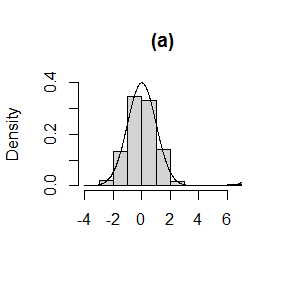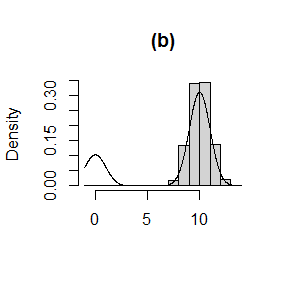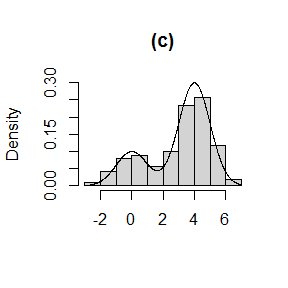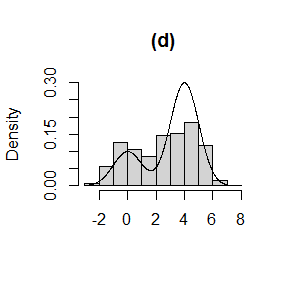# When can rust be used?

#### 2020-09-02

The generalized ratio-of-uniforms can be used to simulate from a wide range of $$d$$-dimensional multivariate probability densities, provided that $$d$$ is not so large that its efficiency is prohibitively low (see the vignette Introducing rust). However, there are conditions that the target density $$f$$ must satisfy for this method to be applicable. This vignette considers instances when these conditions do not hold and suggests strategies that may overcome this difficulty. Although the ratio-of-uniforms method can be used to simulate from multimodal densities, currently rust is designed to work effectively with unimodal densities. This vignette illustrates this using a simple 1-dimensional example.

## Conditions on $$f$$

The generalized ratio-of-uniforms method is an acceptance-rejection type of algorithm. It can only be applied to densities for which its acceptance region can be enclosed within a bounding region of finite volume from which it is simple to simulate, usually a cuboidal bounding box. For a $$d$$-dimensional density $$f(x)$$ the bounding box (if it exists) is the $$(d+1)$$-dimensional set $$\{ 0 < u \leq a(r), \, b_i^-(r) \leq v_i \leq b_i^+(r), \, i = 1, \ldots, d \}$$, where $\begin{eqnarray} a(r) &=& \sup_\chi \, f(x)^{1 / (r d + 1)}, \\ b_i^-(r) &=& \inf_{\chi_i^-} \, x_i \, f(x)^{r / (r d + 1)}, \\ b_i^+(r) &=& \sup_{\chi_i^+} \, x_i \, f(x)^{r / (r d + 1)}, \end{eqnarray}$ $$x =(x_1, \ldots, x_d)$$, $$\chi \subseteq \mathbb{R}^d$$, $$\chi_i^- = \{ x \in \chi, x_i \leq 0 \}$$ and $$\chi_i^+ = \{ x \in \chi, x_i \geq 0 \}$$. See the vignette Introducing rust for more details.

For a given value of the non-negative tuning parameter $$r$$ we need $$f(x)$$ and $$x_i ^ {r d + 1} f(x) ^ r, i = 1, \ldots, d$$ to be bounded. If $$f(x)$$ is unbounded then we could use a transformation of variable to obtain a density that is bounded. For bounded $$f(x)$$ one or more of $$x_i ^ {r d + 1} f(x) ^ r, i = 1, \ldots, d$$ can be unbounded if $$f(x)$$ has heavy-tails. Again, we could use a transformation of variable to avoid this problem. For this issue the value of $$r$$ matters and we may be able to achieve boundedness if a sufficiently large value of $$r$$ is used. In rust $$r = 1/2$$ is used by default because this is optimal in the Gaussian case. For heavy-tailed densities $$r$$ needs to be larger, perhaps larger than 1. We consider these strategies in the next two sections.

## Unbounded densities

A simple example of an unbounded density is that of a gamma random variable with a shape parameter that is less than 1. Suppose that $$X \sim \mbox{gamma}(\alpha, 1)$$ and $$\alpha < 1$$. Then the density $$f_X(x)$$ increases without limit as $$x \rightarrow 0$$ from above. Let $$Y = (X^\lambda - 1) / \lambda$$, that is, a Box-Cox transformation (Box and Cox 1964) of $$X$$. If we choose $$\lambda$$ appropriately then the density $$f_Y(y)$$ of $$Y$$ is bounded. rust has functions for selecting a suitable value of $$\lambda$$ in a somewhat automatic way (the user needs to specify a range of values (min_phi, max_phi) over which to perform the calculations). In the gamma(0.1, 1) case below a value of $$\lambda$$ that is close to 0 is suggested. The plot on the right shows that this does the trick.

library(rust)
alpha <- 0.1
max_phi <- qgamma(0.999, shape = alpha)
ptr_gam <- create_xptr("logdgamma")
lambda <- find_lambda_one_d_rcpp(logf = ptr_gam, alpha = alpha,
max_phi = max_phi)
# Box-Cox transformation parameter
#>  0.5089059
coag2$pa #>  0.3059039 ## Multimodal densities Consider the simple bimodal univariate density produced by a mixture of N(0,1) and N($$m$$, 1) densities, with probability $$p$$ that a value comes from the first component. In principle the generalized ratio-of-uniforms can be used to sample from this density but this relies on the a bounding box being found that includes the entire acceptance region. Currently, ru (and ru_rcpp) search for bounding box parameters in a way that is designed to work well when the density is unimodal. The following examples demonstrate that currently ru isn’t guaranteed to find a suitable bounding box for multimodal densities. normal_mixture <- function(x, mu, p) { return(log(p * dnorm(x) + (1 - p) * dnorm(x, mean = mu))) } res1 <- ru(logf = normal_mixture, mu = 10, p = 0.25, init = -1, n = 1000) plot(res1, main = "(a)") res2 <- ru(logf = normal_mixture, mu = 10, p = 0.25, init = 11, n = 1000) plot(res2, main = "(b)") res3 <- ru(logf = normal_mixture, mu = 4, p = 0.25, init = 5, n = 1000) plot(res3, main = "(c)") res3$pa
#>  0.5473454
res4 <- ru(logf = normal_mixture, mu = 4, p = 0.25, init = -1, n = 1000)
plot(res4, main = "(d)")In (a), using the initial value init = -1` means that the smaller of the two modes is found in the search for $$a(r)$$. As a consequence most of the acceptance region for the other component of the mixture is not contained in the bounding box and this component is effectively missing from the sample produced. Case (b) is similar but the larger of the two modes is found. In (c) and (d) the two components are closer, in the sense that the component distributions overlap to a greater degree. In (c) the larger mode is found, the bounding box contains the entire acceptance region and a valid sample is produced. In (d) the smaller mode is found and much of the acceptance region corresponding to the other component is not included in the acceptance region.

A future release of rust will include an option to employ a more extensive search for the bounding box parameters so that some simple multimodal densities can be accommodated. However, multimodality will tend to reduce the probability of acceptance. In example (c) above it is reasonable (approximately 0.55) but as the number of modes and/or dimensions of the density increase the probability of acceptance will decrease.